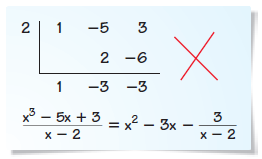#### MAT-HS.A-APR.02

 MAT-HS Targeted Standards(A) Concept: Algebra(SSE) Domain: Arithmetic with Polynomials and Rational ExpressionsCluster: Perform arithmetic operations on polynomials MAT-HS.A-APR.02 Apply the Remainder Theorem.

## Student Learning Targets:

### Knowledge Targets

• I can use the fact that a is a zero of a polynomial.

• I can
• I can

### Skills (Performance) Targets

• I can apply the Remainder Theorem to a polynomial.

• I can
• I can

## Alg II Solve Polynomial Equations Proficiency Scale

Score   Description Sample Activity

4.0

In addition to Score 3.0, the student demonstrates in-depth inferences and applications regarding more complex material that go beyond end of instruction expectations.
 Describe and correct the error in using synthetic division to divide x3-5x+3 by x-2.3.5 In addition to Score 3.0 performance, the student demonstrates in-depth inferences and applications regarding the more complex content with partial success.

3.0

(proficient)

The student can:
• use and apply the remainder theorem/synthetic division to find the zeros of a polynomial function, real and imaginary.
• use and apply long division/synthetic division to find the zeros of a polynomial function.
The student exhibits no major errors or omissions.

Find the zeros:

f(x)= x3-10x2+19x+30

f(x)=2x5+3x4-30x3-57x2-2x+24

f(x)=x3-4x2+25x-100
2.5 The student demonstrates no major errors or omissions regarding the simpler details and processes (Score 2.0 content) and partial knowledge of the more complex ideas and processes (Score 3.0 content).

2.0

(progressing)

There are no major errors or omissions regarding the simpler details and processes as the student can:
• recognize and recalls specific terminology, such as:
• factors
• zeros
• imaginary/complex numbers
• synthetic division or long division
• fundamental theorem of Algebra
• possible zeros (p/q)
• use synthetic division to divide polynomials.
• can perform operations with complex numbers.

However, the student exhibits major errors or omissions regarding the more complex ideas and processes.

Synthetically divide to find the remaining zeros.

(6x3-19x2+16x-4)(x-2)

(2x4+7x3-4x2-27x-18)(x+3)
1.5 The student demonstrates partial knowledge of the simpler details and processes (Score 2.0 content) but exhibits major errors or omissions regarding the more complex ideas and procedures (Score 3.0 content).

1.0

(beginning)

With help, the student demonstrates a partial understanding of some of the simpler details and processes (Score 2.0 content) and some of the more complex ideas and processes (Score 3.0 content).
0.5 With help, the student demonstrates a partial understanding of some of the simpler details and processes (Score 2.0 content) but not the more complex ideas and processes (Score 3.0 content).
0.0 Even with help, the student demonstrates no understanding or skill.

## Resources

 Web Vocab